In :
from pystencils.session import *

import shutil


# Plotting and Animation¶

## 1) Stencils¶

This section shows how to visualize stencils, i.e. lists of directions

In :
stencil_5pt = [(0, 0), (1, 0), (-1, 0), (0, 1), (0, -1)]
plt.figure(figsize=(2, 2))
ps.stencil.plot(stencil_5pt)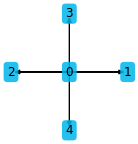By default the indices are shown the arrow endpoints. Custom data can also be plotted:

In :
plt.figure(figsize=(2, 2))
ps.stencil.plot(stencil_5pt, data=[2.0, 5, 'test', 42, 1])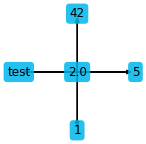The stencil can also be given as an expression in a single field

In :
f = ps.fields("f: [2D]")
c = sp.symbols("c")
expr = f[0, 0] + 2 * f[1, 0] + c * f[-1, 0] + 4 * f[0, 1] - c**2 * f[0, -1]
plt.figure(figsize=(2, 2))
ps.stencil.plot_expression(expr)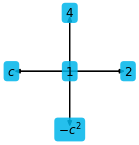There is also a function to simply plot sympy expressions depending on one variable only:

In :
x = sp.symbols("x")
plt.figure(figsize=(4, 4))
plt.sympy_function(x**2 - 1, x_values=np.linspace(-2, 2));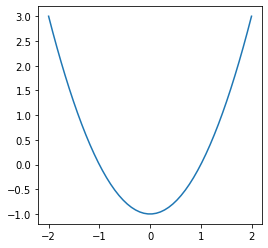Three dimensional stencils can be visualized in two different ways

In :
stencil_7pt = [(0, 0, 0), (1, 0, 0), (-1, 0, 0), (0, 1, 0), (0, -1, 0), (0, 0, 1), (0, 0, -1)]
ps.stencil.plot(stencil_7pt, data=[i for i in range(7)])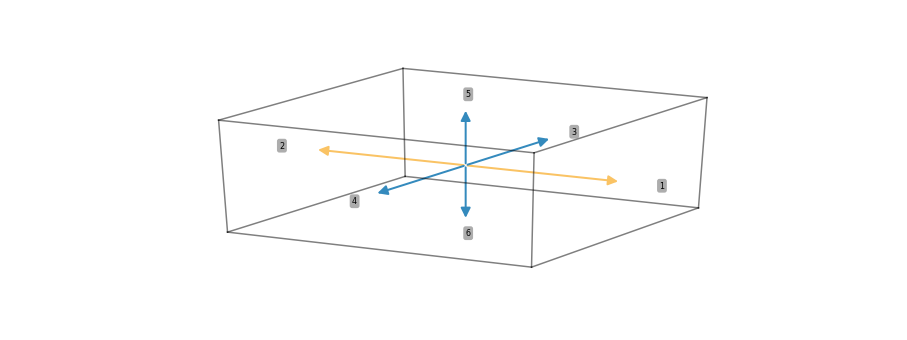This kind of plot works well for small stencils, for larger stencils this gets messy:

In :
stencil_27pt = [(i, j, k) for i in (-1, 0, 1) for j in (-1, 0, 1) for k in (-1, 0, 1)]
ps.stencil.plot(stencil_27pt, data=[i for i in range(27)])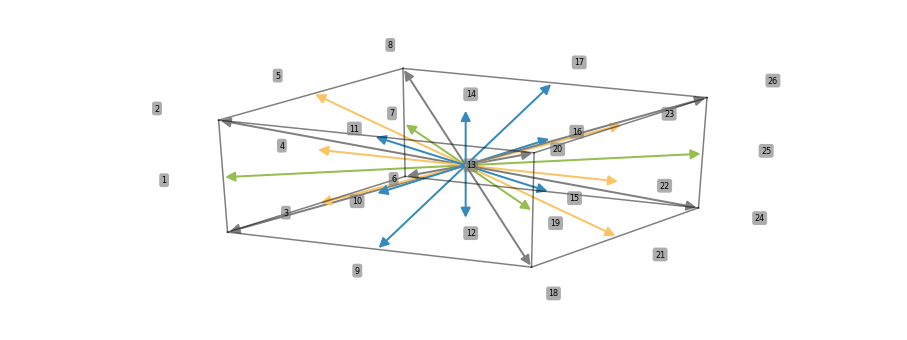Adding slice=True shows the three projections of the stencils instead

In :
ps.stencil.plot(stencil_27pt, data=[i for i in range(27)], slice=True)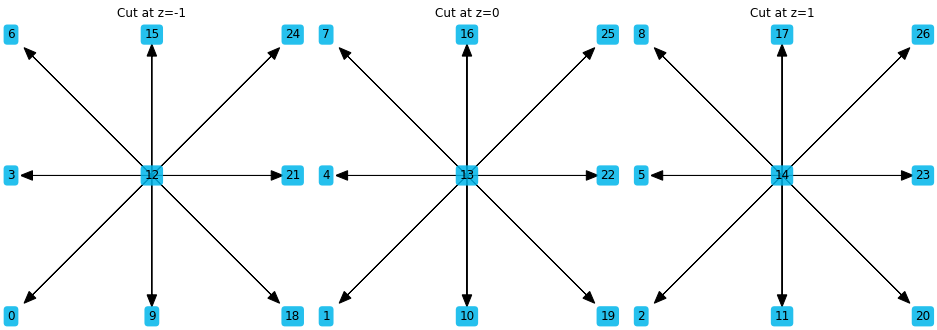## 2) Scalar fields¶

pystencils also comes with helper functions to plot simulation data. The plotting functions are simple but useful wrappers around basic Matplotlib functionality. pystencils imports all matplotlib functions as well, such that one can use

import pystencils.plot as plt

import matplotlib.pyplot as plt

The session import at the top of the notebook does this already, and also sets up inline plotting for notebooks.

This section demonstrates how to plot 2D scalar fields. Internally imshow from matplotlib is used, however the coordinate system is changed such that (0,0) is in the lower left corner, the $x$-axis points to the right, and the $y$-axis upward.

The next function returns a scalar field for demonstration

In :
def example_scalar_field(t=0):
x, y = np.meshgrid(np.linspace(0, 2 * np.pi, 100), np.linspace(0, 2 * np.pi, 100))
z = np.cos(x + 0.1 * t) * np.sin(y + 0.1 * t) + 0.1 * x * y
return z


To show a single scalar field use plt.scalar_field:

In :
arr = example_scalar_field()
plt.scalar_field(arr)
plt.colorbar();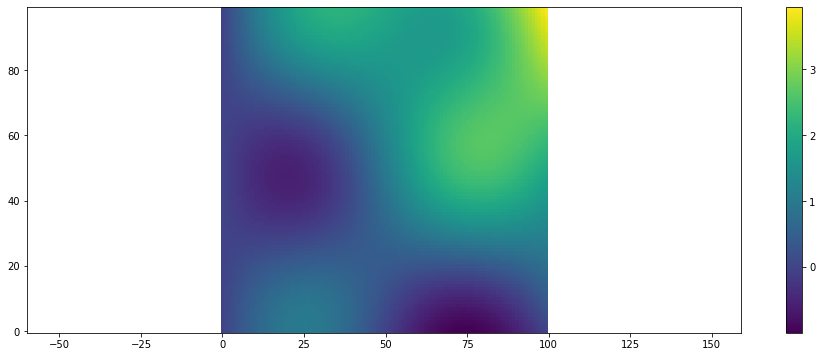A similar wrapper around counter from matplotlib

In :
plt.scalar_field_contour(arr);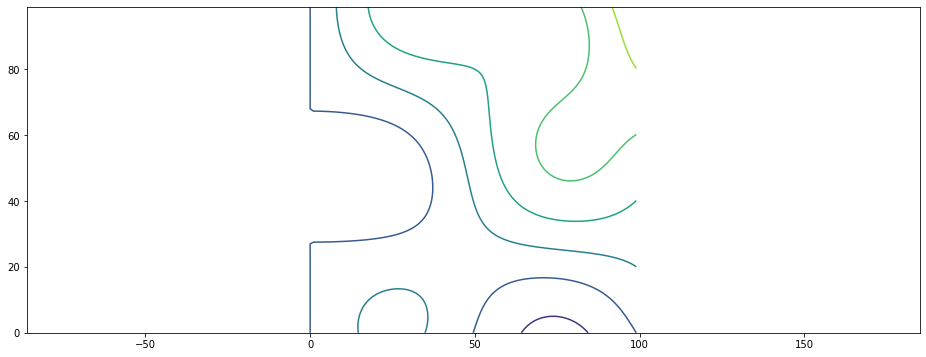A 3D surface plot is also possible:

In :
plt.scalar_field_surface(arr)

Out:
<mpl_toolkits.mplot3d.art3d.Poly3DCollection at 0x7f38908f9828>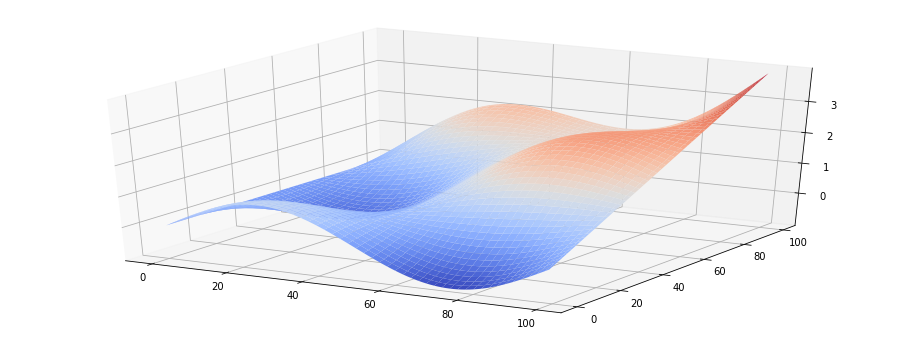For simulation results one often needs visualization of time dependent results. To show an evolving scalar field an animation can be created as shown in the next cell

In :
t = 0
def run_func():
global t
t += 1
return example_scalar_field(t)

In :
if shutil.which("ffmpeg") is not None:
plt.figure()
animation = plt.scalar_field_animation(run_func, frames=60)
ps.jupyter.display_as_html_video(animation)
else:
print("No ffmpeg installed")

Out:

Another way to display animations is as an image sequence. While the run_func i.e. the simulation is running the current state is then displayed as HTML image. Use this method when your run_func takes a long time and you want to see the status while the simulation runs. The frames parameter specifies how often the run function will be called.

In :
plt.figure()
animation = plt.scalar_field_animation(run_func, frames=30)
ps.jupyter.display_as_html_image(animation)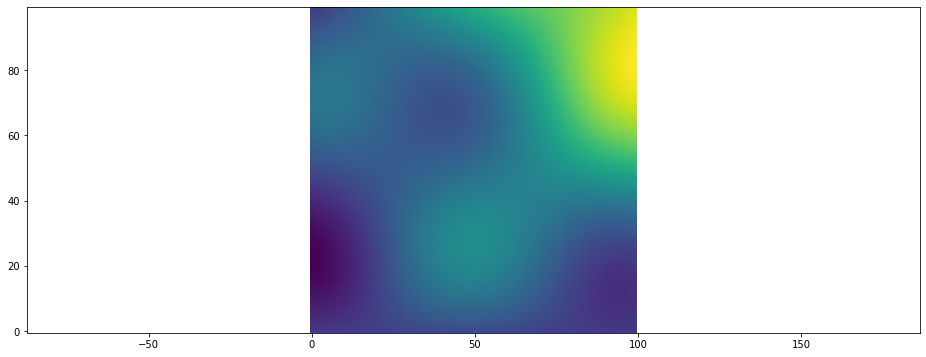For surface plots there is also an animated version:

In :
if shutil.which("ffmpeg") is not None:
animation = plt.surface_plot_animation(run_func, frames=60)
ps.jupyter.display_as_html_video(animation)
else:
print("No ffmpeg installed")

Out:

## 3) Vector Fields¶

pystencils provides another set of plotting functions for vector fields, e.g. velocity fields. They have three index dimensions, where the last coordinate has to have 2 entries, representing the $x$ and $y$ component of the vector.

In :
def example_vector_field(t=0, shape=(40, 40)):
result = np.empty(shape + (2,))
x, y = np.meshgrid(np.linspace(0, 2 * np.pi, shape), np.linspace(0, 2 * np.pi, shape))
result[..., 0] = np.cos(x + 0.1 * t) * np.sin(y + 0.1 * t) + 0.01 * x * y
result[..., 1] = np.cos(0.001 * y)
return result


Vector fields can either be plotted using quivers (arrows)

In :
plt.figure()
data = example_vector_field()
plt.vector_field(data, step=1);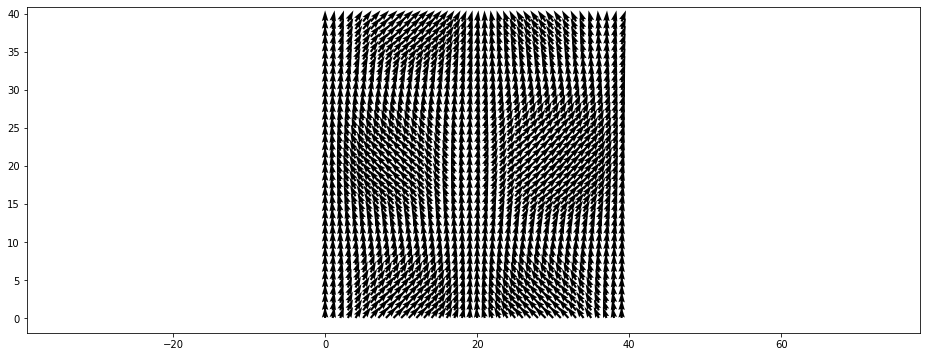The step parameter can be used to display only every $n$'th arrow:

In :
plt.figure()
plt.vector_field(data, step=2);